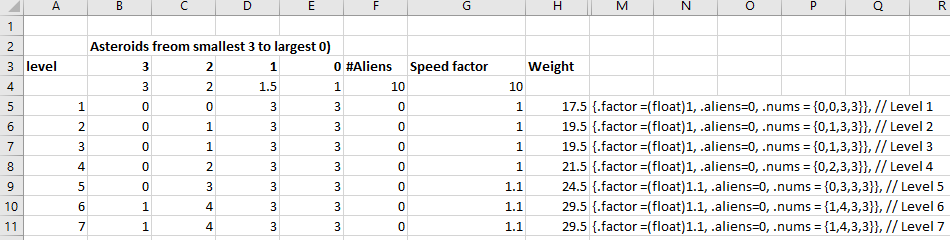## Using Excel for level designIn the asteroids game and shortly in my MatchThree game, I’ll be creating a level data array of struct. This has a struct for each level containing a count of particular features for that level.

This is the struct and array for a level in asteroids.

``````struct level {
int nums; // how many of each size of asteroid
int aliens; // how many aliens
float factor; // from 1.0 to 1.5 - multiply asteroid speed by this
};

struct level levels;``````

This is the first 3 levels and level 50.

``````#include "levels.h"

struct level levels = {
{ .factor = (float)1,.aliens = 1,.nums = { 0,0,3,3 } }, // Level 1
{ .factor = (float)1,.aliens = 0,.nums = { 0,1,3,3 } }, // Level 2
{ .factor = (float)1,.aliens = 0,.nums = { 0,1,3,3 } }, // Level 3
..
{ .factor = (float)1.5,.aliens = 3,.nums = { 4,4,5,5 } } // Level 50
``````

I didn’t type any of this in. Instead, I created that spreadsheet above. It’s easy to work out difficulty levels in column H.  The formula that calculates this is ins this on row 5. If you don’t know Excel, the \$ in the factors means that as you copy this into successive row, it keeps the \$ row value constant.

```=(B5*B\$4)+(C5*C\$4)+(D5*D\$4)+(E5*E\$4)+(F5*F\$4)+(G5*G\$4)   - Row 5

=(B6*B\$4)+(C6*C\$4)+(D6*D\$4)+(E6*E\$4)+(F6*F\$4)+(G6*G\$4) - Row 6```

So you can see its multiplying the values in rows 5 6 etc by the values in row 4.  Having put that in place I could tinker with the values in rows 5,6 etc to make the difficulty level increase roughly at the same pace. The difficulty level for level 50 is 77.5.

This is what the Excel formula looks like to generate the C code, it’s in cell M5 in the spreadsheet and then copied and pasted down.

`="{.factor =(float)"&G5&", .aliens="&F5&", .nums = {"&B5&","&C5&","&D5&","&E5&"}}, // Level "&A5`

and this is what it looks like. C code that can be copied and pasted directly. It even includes the comment for the level number!

 `{.factor =(float)1, .aliens=0, .nums = {0,0,3,3}}, // Level 1`

Creating a spreadsheet and C code from it this way saved a lot of typing but let me quantify the numerical difficulty which increases from 17.5 on level 1 to 77.5 on level 50. There’s no meaning to this value, it’s just a calculation.